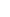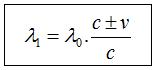25. WHERE AND WHEN DOES DOPPLER SHIFT OCCUR?We started the topic (c+v) (c-v) mathematics with Doppler Shift. Doppler Shift is such an important topic that words fall short trying to explain its significance. We have seen its results so far; now, we need to understand how it is formed. Doppler Shift cannot occur without having a mechanism or a foundation in the nature that leads to Doppler Effect. How does an electromagnetic wave know the speed of its arrival target? How does it know its arrival target is in motion? How can it stabilize its speed as c relative to its arrival target? Questions to be asked are not limited to these. There are weightier questions, too. Lets have a look at the wavelength change equation in Doppler Shift.Mass sizes of objects dont have importance for the equation. The fact that two objects are as big as celestial bodies or as small as molecules doesnt change the equation. It can also be seen from the equation that Doppler Shift is independent of distance. Even in a situation when the distance between two objects is hundreds of light years, the equation doesnt change. In both aspects, Doppler Shift shares a striking similarity with Law of Universal Gravitation and Coulombs Law. In terms of these laws mass size and the distance between have no importance.Different from the Law of Universal Gravitation and Coulombs Law, Doppler Shift equation carries quite a big and important information in itself and it is this: Doppler Shift equation clearly shows that the wavelength change occurs at the moment of signal emission. This is truly tremendous information. Lets elaborate on and analyze how we are faced with something now.Lets talk about any star that is a few million years away from our world. By measuring the wavelength of the light coming from the star, we find out the wavelength change and, as a result, we determine whether the star is approaching us or moving away from us. If we are careful, we will see that there are only three options for the place of the wavelength change.  1) Wavelength changes at the moment of signal emission. 2) Signal wavelength changes in a way during the travel of the signal after its emission. 3) Wavelength doesnt change, but it is perceived as if it has changed at the arrival point.Lets eliminate our options one by one.Option 2 is highly irrational. Why would an electromagnetic wave change its wavelength on its own after it has already set out and while it is traveling on its own path? Where and when this change will take place during the travel? Will the signal wavelength lengthen or shorten? Why does it form wavelength change in a way that it gives Doppler Equation? How can an electromagnetic wave do this without knowing where it is going? As can be seen, there are so many confusions and so much obscurity in this option. Everyone is free to think through this option, but my advice is to eliminate this option.Although option 3 has a logical background, it is completely against the realities of physics. The wavelength doesnt change but it is perceived as if it has changed at the arrival target. For such an option to be true, the signal speed coming to the arrival target must be c±v relative to the reference system of the arrival target itself (in case the source and the target are moving away from each other (c+v) and when they are approaching each other (c-v)). Only in this case can a difference be measured at the reference system of the arrival target; this is a very clear result. Now that we know for sure that, relative to the reference system of the arrival target, the speed of an INCOMING signal coming to the target itself is always c, we eliminate this option. This option doesnt even have a little chance.As can be seen, we are pushed towards option 1 as a result of a short analysis. Signal wavelength change happens at the time of the signal emission. Then, after this stage, we need to look for answers to the questions that option 1 included in it. And we have quite serious questions that need to be answered. The toughest of all these questions are: How can an electromagnetic wave know its arrival target at the moment of the emission?  How is the information that the arrival target is in motion conveyed to the source that emits the electromagnetic wave? How can an electromagnetic wave adjust its speed as c relative to its arrival target if the arrival target is in motion relative to the source? How can a source whose frequency is f0 and wavelength λ0 as its factory setting from its manufacture emit wavelengths over different wavelengths?  If the questions above can be answered, how can a source do these even when there are unbelievable distances thousands, millions, and even billions of light years  between the source and the target?  Let me answer them at once.  Errrrrrrr,........ umm....... *cough*  ouhhhhu,.well..........., I mean.................,  *cough*, errrrrrrrr.........., *cough*,......, ouhhuu..........., *COUGH*.BREAK TIME. We are giving a mandatory break at this point. We need more information to be able to answer these questions.